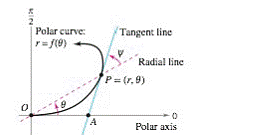Chapter 10.4, Problem 106E

Chapter
Section
Textbook Problem

Proof Prove that the tangent of the angle ψ ( 0 ≤ ψ ≤ π / 2 ) between the radial line and the tangent line at the point (r, θ ) on the graph of r = f ( θ ) (see figure) is given by tan ψ = | r d r / d θ |q

To determine

To prove:

The expression tanψ=|rdrdθ| if the tangent of angle ψ(0ψπ2) between the radial line and the tangent line at the point (r,θ) on the graph of r=f(θ) is given..

Explanation

Given:

The given function is r=f(θ).

and tanψ=|rdrdθ| and the graph is shown as below:

Formula used:

The slope of tangent line at point (r,θ) is,

dydx=dydθdxdθ=f(θ)cosθ+f(θ)sinθf(θ)sinθ+f(θ)cosθ.

Use the trigonometric formula,

tan(x+y)=tanx+tany1tanxtany

Proof:

Since the slope of the tangent line at point (r,θ) (r,θ) is

dydx=f(θ)cosθ+f(θ)sinθf(θ)sinθ+f(θ)cosθ …… (1)

From the graph, it can be seen that the slope of the tangent line through A and P is,

tan(θ+ψ)=tanθ+tanψ1tanθtanψ

Since, tanθ=sinθcosθ,

tan(θ+ψ)=sinθ+cosθtanψcosθsinθtanψ …… (2)

Thus equate equation (1) and equation (2) and then cross-multiply them,

f(θ)cosθ+f(θ)sinθf(θ

Still sussing out bartleby?

Check out a sample textbook solution.

See a sample solution

The Solution to Your Study Problems

Bartleby provides explanations to thousands of textbook problems written by our experts, many with advanced degrees!

Get Started

Find more solutions based on key concepts